summary of site-wide JavaScript functionalityUnited States-English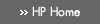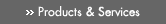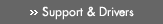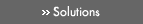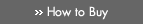» Contact HPSearch:More optionsManualTechnical documentation - EnglishAll of HP USHP-UX Reference > M# math(5)

HP-UX 11i Version 3: February 2007»

## Technical documentation» Feedback## » Index## NAME

math — math functions, constants, and types

## SYNOPSIS

#include <math.h>

## DESCRIPTION

This file contains declarations of all the functions in the Math Library (described in Section 3M).

For HP Integrity servers, included in a compilation under the -fpwidetypes option, this file defines the types

extended

The Integrity server 80-bit double-extended type.

An IEEE 754-compliant, 128-bit floating-point type. On HP-UX, quad is a synonym for long double.

It defines the types

float_t

A floating type, at least as wide as float. For PA-RISC, float_t is float. For Integrity servers, if FLT_EVAL_METHOD equals 0, float_t is float; if FLT_EVAL_METHOD equals 1, float_t is double; if FLT_EVAL_METHOD equals -2, float_t is extended.

double_t

A floating type, at least as wide as double. For PA-RISC, double_t is double. For Integrity servers, if FLT_EVAL_METHOD equals 0 or 1, double_t is double; if FLT_EVAL_METHOD equals -2, double_t is extended.

For Integrity servers, the value of FLT_EVAL_METHOD is 0, 1, or -2, according to the use of the compiler option -fpeval=float(default), -fpeval=double, or -fpeval=extended.

It defines the following constants, which, for an Integrity server, may be used to initialize statics and aggregates:

NAN

A quiet NaN (Not-a-Number) value (type float).

INFINITY

A positive infinity value (type float).

HUGE_VAL

The maximum value (type double) representable in the double type (IEEE positive infinity).

HUGE_VALF

The maximum value (type float) representable in the float type (IEEE positive infinity).

HUGE_VALL

For Integrity servers only, the maximum value (type long double) representable in the long double type (IEEE positive infinity).

HUGE_VALW

For Integrity servers only, the maximum value (type extended) representable in the extended type (IEEE positive infinity). To use HUGE_VALW compile with the -fpwidetypes option.

HUGE_VALQ

For Integrity servers only, equivalent to HUGE_VALL. To use HUGE_VALQ compile with the -fpwidetypes option.

It defines the following integer constants which give special-case values returned by the ilogb() functions:

FP_ILOGB0

Returned by ilogb() if its argument is zero.

FP_ILOGBNAN

Returned by ilogb() if its argument is a NaN.

For Integrity servers, it defines the following integer constants which identify the error handling method supported by <math.h> functions:

MATH_ERRNO

Indicates support for the ISO/IEC C99 errno specification.

MATH_ERREXCEPT

Indicates support for the ISO/IEC C99 exception flag specification.

math_errhandling

Defined to be MATH_ERRNO if the +Olibmerrno compiler option (not the default) is used, or defined to be MATH_ERREXCEPT otherwise.

It defines the following mathematical constants (type double) for user convenience:

M_E

The base of natural logarithms (e).

M_LOG2E

The base-2 logarithm of e.

M_LOG10E

The base-10 logarithm of e.

M_LN2

The natural logarithm of 2.

M_LN10

The natural logarithm of 10.

M_PI

The ratio of the circumference of a circle to its diameter. (There are also several fractions of π, its reciprocal, and its square root: M_PI_2, M_PI_4, M_1_PI, M_2_PI, and M_2_SQRTPI).

M_SQRT2

The positive square root of 2.

M_SQRT1_2

The positive square root of 1/2.

For the definitions of various machine-dependent constants, see the description of the <values.h> header file.

To use one of the types extended or quad, or one of the macros HUGE_VALW or HUGE_VALQ, compile with the -fpwidetypes option.

## FILES

/usr/include/math.h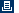Printable versionPrivacy statement Using this site means you accept its terms Feedback to webmaster© 1983-2007 Hewlett-Packard Development Company, L.P.